## User-Defined Functions – Writing Your Own Functions in Excel

There are over 500 built-in functions in Excel but yet you may have trouble finding one that suits your need. Are there calculations that you do on a regular basis over and over again? And you are tired of typing in that same formula in again and again every time. You can save time by writing your very own user-defined functions in Excel.

Before we start, we need to make sure the Developer tab is enabled in Excel. If you don’t see a Developer tab at the top, it’s simple – have a look at How To Enable Macro/Visual Basic for Application (VBA):If you can see the Developer tab, then let’s get started! We will first go through a simple example of creating a function that calculates the area of a circle. And then we will go through another example which is more complex and uses a loop.

## Creating Your Very Own Functions

### 1. Open Visual Basics

You can open Visual Basics by going to Developer Tab > Visual Basics: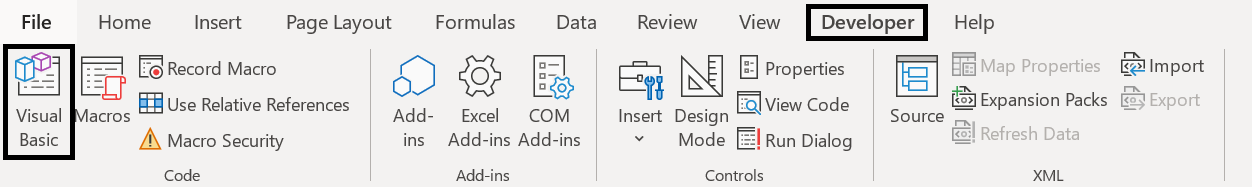Or you can press Alt + F11.

### 2. Create a New Module

At the top, click on Insert > Module: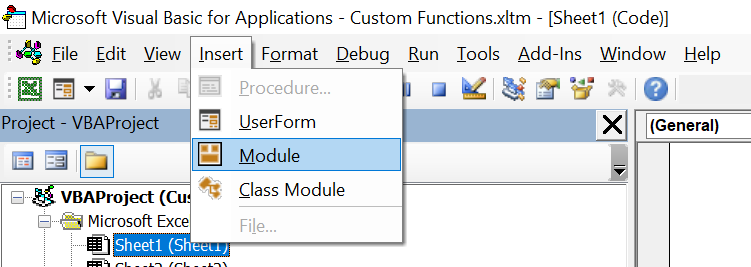### 3. Name of Function and Required Field(s)

#### 3.1. Unique Name for the Function

Now it’s time to think of a unique name for the function and what variables or fields should be required. It is best practice to give the function a name that is relevant to its purpose. For example, it’ll be very confusing to others who use the spreadsheet if you name the function “Bob”. And it’ll be confusing for yourself. A few months later you might come back and wonder what the function “Bob” is supposed to calculate. In this example we will create a user-defined function that calculates the area of a circle so we will call the function “AreaOfCircle”.

You also want the name to be unique. Because Excel already has over 500 built-in functions, you won’t want one that is already used by Excel. In fact, if you do, your user-defined functions will not work. Excel’s built-in existing functions will take place over your user-defined functions.

#### 3.2. Variables and Fields

Technically not every function requires variables. It probably won’t be of much use but you can create a function that returns a constant value every time. For example you can leave the variables blank and have the function AreaOfCircle = 2 in the module. In which case, AreaOfCircle() will always return 2. But for example if we want to calculate area of a rectangle then the variables will be length and width.

In this scenario, what variables are required in order to calculate the area of a circle? Since A = πr2, the only variable is r – the radius. In the empty module, enter the following:

And in our case: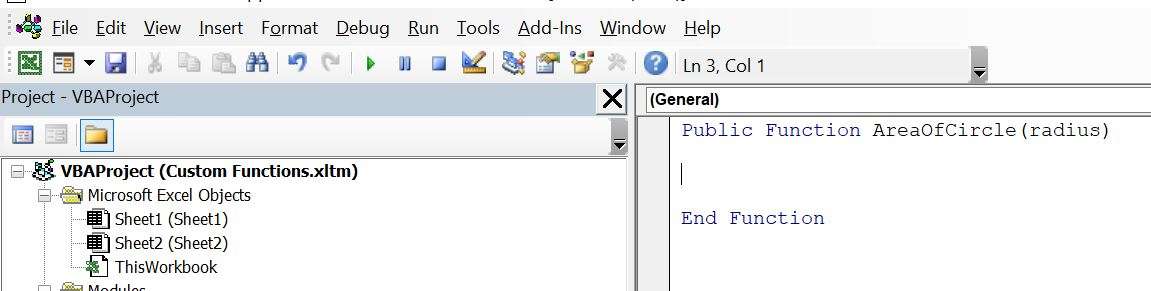### 4. Write Your Formula and Calculation

This is of course the crux of the function. What is your function? What would you like Excel to calculate for you with this new function? To stick with our example above, we want to calculate the area of a circle: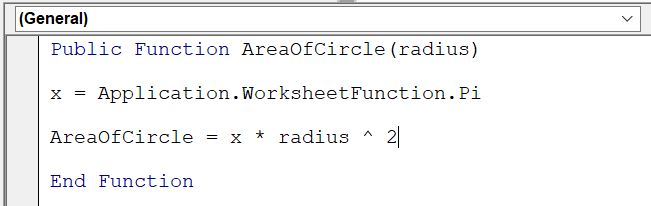We first set a new article x to be the value of Pi. And then we set AreaOfCircle to equal x * radius ^ 2 which is equivalent to A = πr2. In this case we set x as Pi.

### 5. Let’s Check Our Work!

Go back to our Excel sheet. Click on any cell and start typing “=” and the name of your function. You should be able to see it: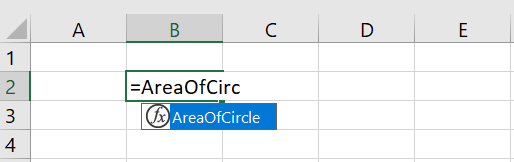Once you see that your function is there, let’s check the calculation. The easiest way is to use this function in one cell and then put in the exact same formula in the cell underneath it manually with the same variable(s). Check out below: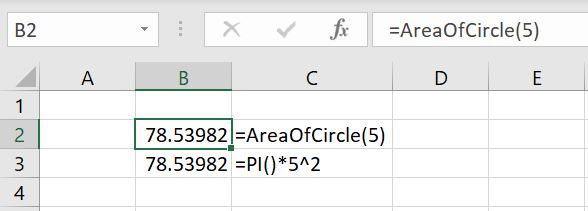The results are the same which is good. Test out a few more variables to make sure the function is working as intended.

### 6. (Optional) Setting Variables Using IF Statements

Now that our function is working, is there a way to improve it? This part is optional. Depending on what your function is, in most cases there should be parameters around what your variables could be. For example in some cases, the variables should only be integers. And in the case of a circle, the radius should only be a positive number. It can be a decimal but it certainly does not make sense to have a negative radius.

To do this:

Before defining the AreaOfCircle function, add an IF function. If the radius variable entered is less than 0, the function will return “Invalid variable entered”. In all other situations, calculate the area of circle.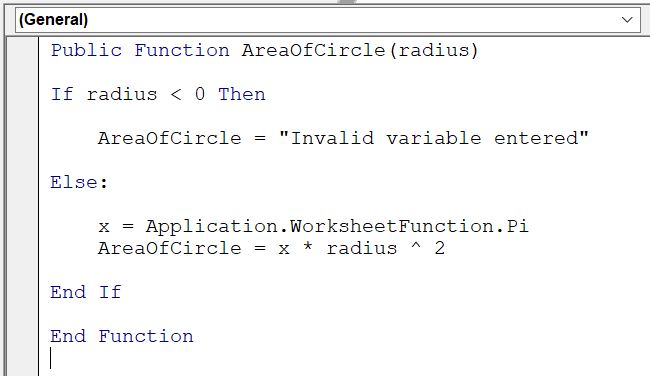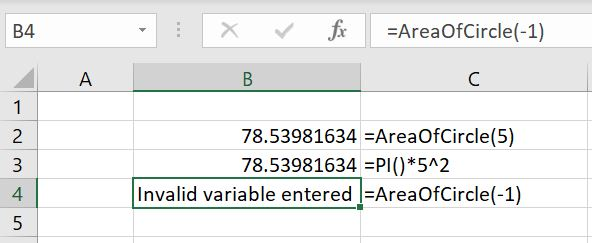In these cases, notifying the user an invalid variable was entered would most likely be more useful than actually calculating the area of circle with a negative 1 radius.

Now let’s move onto a more complex example which involves a loop in the user-defined function

## Creating a User-Defined Function with Loop

When we were writing the article for COUNT functions, it got us wondering if, given a specified range of cells, there was a way to count the number of cells containing data that has more than x number of characters inside. Well let’s write our own: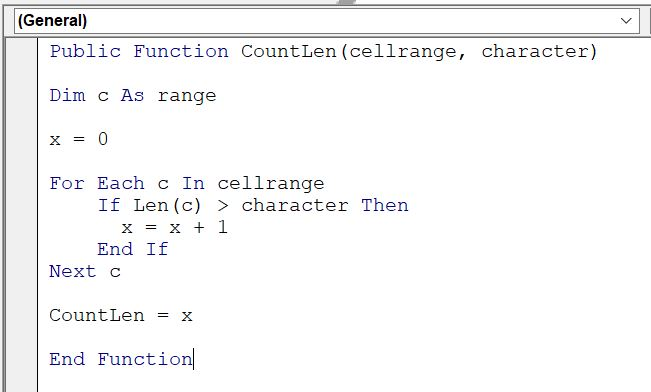To put this into words:

• We’ve called this new function “CountLen” and there are two variables required by the user every time: 1) the range of cells to count in (cellrange) 2) how many characters should be in the cell in order for the function to count the cell as meeting the condition (character)
• First we create a new variable x and we set it as 0 at first. And for each cell in the range of cells specified (cellrange), if the number of character is greater than what we’ve specified in the second variable (character) then Excel will add 1 to x every time. After all the cells have been looped through, CountLen will return the value of x.

Here’s an example below. In A2:A15, we’ve entered a bunch of random data. Let’s test this out with different variables to check if our function is working. Note that in the function, we’ve decided to set the function to check if the number of characters in each cell is greater than second variable specified, not greater than or equal to. Hence if we set the second variable as 2, Excel will only count if the number of characters is 3 or more: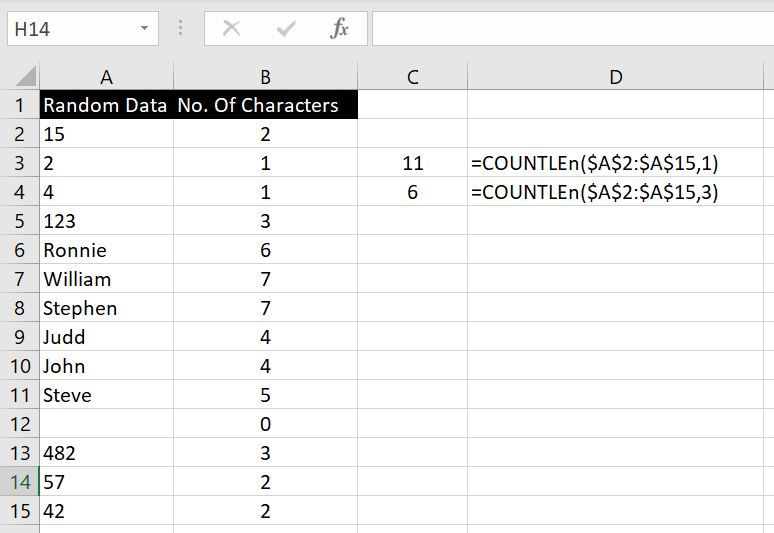To check our results, we created Column B which counts the number of characters in corresponding cell in Column A with the LEN function. There are 11 cells in A2:A15 that have more than one character in them, and there are 6 cells in A2:A15 that have more than 3 characters in them.

Are there any user-defined functions you need help with and want us to go through? Let us know in the comment below and we will keep add to our article to make it more complete.